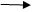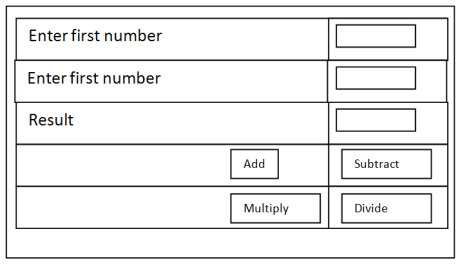Data Contract in WCF services
Ratings:
(4)
Views:342## Arithmetic operations using data contract in WCF servicesCreate a new WCF service with the name example2Change the interface name form I service.cs to I arithematic.cschange the class name from service.cs to cls arthematic.cschange the service file name from service.svc to cls arithematic.svcdouble click on I arithematic.cs & write the following code.In data contract we declare all data fields & properties.To provide data facilities to the services contract we use data contract.Data contract class object reference is used in service contract [Service contract] Public interface I arithematic { [operation contract] Int add (Arithmetic data obj1); [operation contract] Int subtract (arithematic data obj1); [operation contract] Int multiply (arithematic data obj1); [operationcontact] Int divide (arithematic data obj1); } [Data contract} Public class arithematicdata { Int num1, num2, result; [data member] Public int p num1 { Set{ num 1 = value;} Get {return num1:} } [data member] Public int p num 2 { Set {num 2 = value;} Get { return num2:} [Data member] Public int presult { Set { result = value;} Get {return result;} } } Double click on cls arithmetic.cs file in solution explorers write the following code Pulbic class cls arithematic : I arithematic { Public int add (arithematic data obj1) { Obj1. Presult = obj 1.pnum1 + obj 1 .pnum2; return obj1. Presult; } Public int subtract (arithematic data obj1) { Obj1. Presult = obj1. Pnum1 – obj1. Pnum2; return obj1. Presult; } public int multiply (arithmetic data obj1) { obj1. Presutl = Obj1. Pnum1* obj1.pnum2: return obj1. Presult: } Public int divide (arithematic data obj1) { obj1. Presult=obj1. Pnum1/obj1.pnum2; return obj1. Presutl;}} Change in cls arithematic.sve file the service name to cls arithematic à to to web. Config file change the service name to cls arithematic & contract ot I arithmetic à run the application & check the path of cls Arithematic.Svc

## Example to consume the WCF service / creating client component:-Create a new web site with the name “check example2”desing the webpage.add reference to example2 WCF service class i.e., cls arithematic.svc with namespace servie reference name as “SR1”write the following code. Using SR1: Arithematic client objs = new Arithematic client (); Arithematic data objd = new arithematic data (); Protected void btn add – click (---) { objD.pnum1= convert. TOInt 32 (txtnum1.text) objD.pnum2= convert. TOInt 32 (txtnum2.text) objD.pnum = objs. Add (objD); text result. Text = objD. Presult. Tostring(); } Protected void btnsubtruct_ click (=---) { objD.pnum1= convert. TOInt 32 (txtnum1.text) objD.pnum2= convert. TOInt 32 (txtnum2.text) objD.pnum = objs. Add (objD); text result. Text = objD. Presult. Tostring(); } Protected void btndivide – click (---) { objD.pnum1 = convert. Toint32 (textnum1.Text); objD.pnum2 = convert. Toint32 (textnum2.Text); objD.preset = objs. Divide (obj D): txtresult . text = ObjD. Presutlt. Tostring(); }}